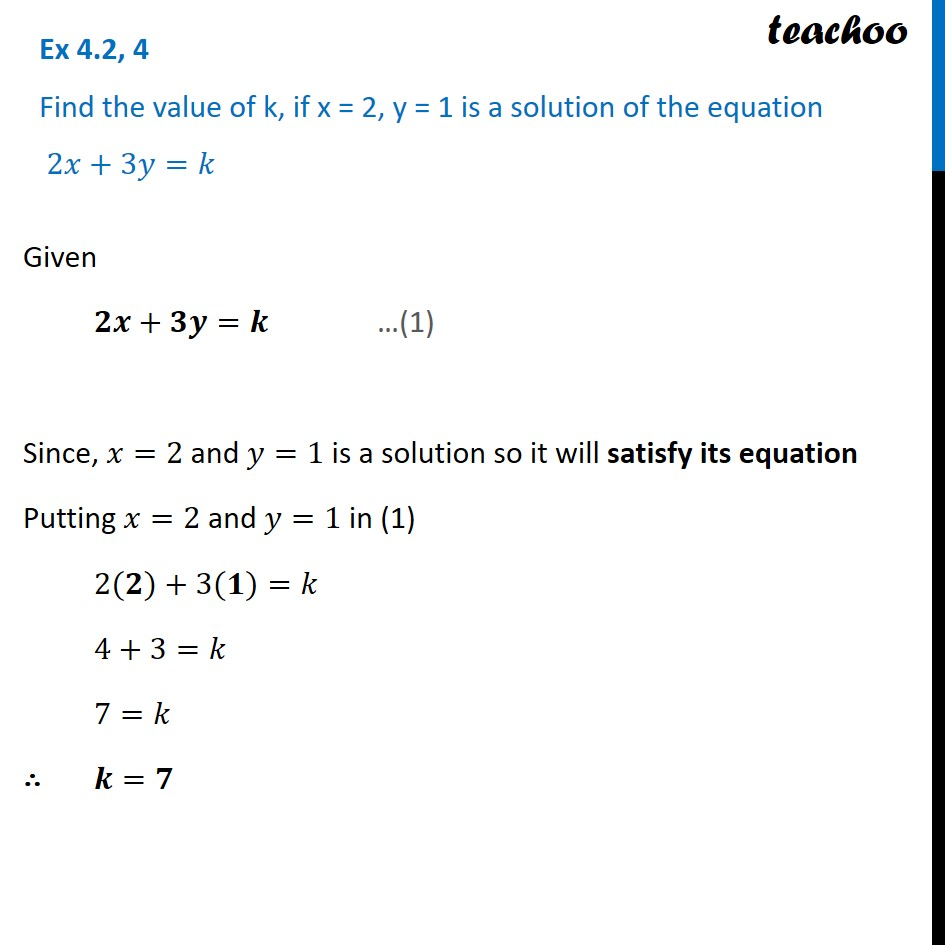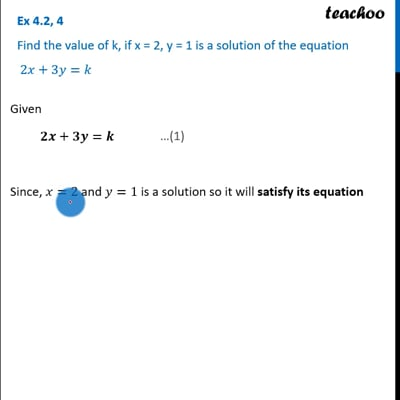Ex 4.2

Chapter 4 Class 9 Linear Equations in Two Variables
Serial order wiseThis video is only available for Teachoo black users

Solve all your doubts with Teachoo Black (new monthly pack available now!)

### Transcript

Ex 4.2, 4 Find the value of k, if x = 2, y = 1 is a solution of the equation 2𝑥+3𝑦=𝑘Given 𝟐𝒙+𝟑𝒚=𝒌 Since, 𝑥=2 and 𝑦=1 is a solution so it will satisfy its equation Putting 𝑥=2 and 𝑦=1 in (1) 2(𝟐)+3(𝟏)=𝑘 4+3=𝑘 7=𝑘 ∴ 𝒌=𝟕 …(1)